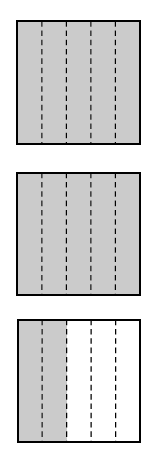### Home > CC1 > Chapter Ch4 > Lesson 4.2.3 > Problem4-71

4-71.

George drew the diagram at right to represent the number $2\frac{2}{5}$. “Look,” said Helena, “This is the same thing as $\frac { 12 } { 5 }$ .” What do you think? Explore this idea in parts (a) through (c) below.

1. Is Helena correct? If so, explain how she can tell that the diagram represents $\frac { 12 } { 5 }$ . If she is not correct, explain why not.

How much does each piece represent?

Since each square is divided into $5$ equal pieces, each piece is equal to one fifth.
There are $12$ shaded pieces, so this diagram represents

2. Draw a diagram to represent the mixed number $3\frac{2}{3}$. How can you write this as a single fraction greater than one?

Use the diagram for part (a) to help you design this diagram.
How many pieces are in a whole? How many do you shade?

To represent this mixed number as a single fraction greater than one, $11$ equal pieces will need to be shaded.
How much of a whole will each piece represent?

3. How can you write $\frac { 7 } { 4 }$ as a mixed number? Be sure to include a diagram in your answer.

• How many whole numbers does this represent?
How many pieces are left over?
Remember to draw a diagram.